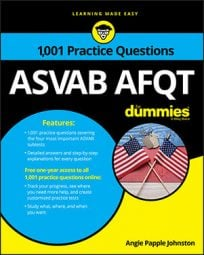##### ASVAB AFQTYou'll find plenty of multi-step problems on the Arithmetic Reasoning subtest on the ASVAB. Keep in mind that the correct answers hinge on paying attention to detail, so you make sure you read every question thoroughly before you formulate your answer!

## Practice questions

1. Luz filled up her 10-gallon gas tank with regular unleaded fuel for \$30. Tony used premium fuel, which costs 25 percent more per gallon, to fill his 10-gallon gas tank. Assuming both of their gas tanks were empty, how much did Tony spend?

A. \$37.75 B. \$3.75 C. \$37.50 D. \$30

2. If one side of a square is increased by 6 cm and an adjacent side is decreased by 2 cm, a rectangle with a perimeter of 28 cm is formed. What is the area of the rectangle?

A. 19 cm2 B. 25 cm2 C. 31 cm2 D. 33 cm2

1. The correct answer is Choice (C).

Write an equation that lets x represent the cost of 1 gallon of unleaded fuel. Ten gallons cost Luz \$30, so

Because premium fuel costs 25 percent more, it costs 125 percent of the price of unleaded fuel, so let 1.25x represent the amount Tony spent per gallon. Unleaded gas costs \$3 per gallon, so figure out how much Tony spent per gallon by replacing x in his equation with 3:

\$3(1.25) = \$3.75

Each gallon of premium fuel costs \$3.75 and Tony bought 10 gallons, so multiply 3.75 by 10 to find out how much Tony spent:

Tony spent \$37.50 on fuel.

2. The correct answer is Choice (D).

This problem requires you to take a few steps to arrive at the answer.

Create a table that lets you visualize what to use in your equation, letting x represent the length of one side of the square:

The problem gives you the perimeter of the rectangle, so use the perimeter formula to find the dimensions — you'll need them to find the rectangle's area. Remember that P = 2l + 2w, where P represents perimeter, l represents length, and w represents width:

Now you know that the original square's sides each measured 5 centimeters. As the problem tells you, one side was increased by 6 centimeters to create the rectangle, while the other was decreased by 2 centimeters. That makes one side 11 centimeters and the other 3 centimeters. You can find the area of the rectangle with the formula A = lw, where A represents the area, l represents the length, and w represents the width:

The rectangle's area is 33 square centimeters.